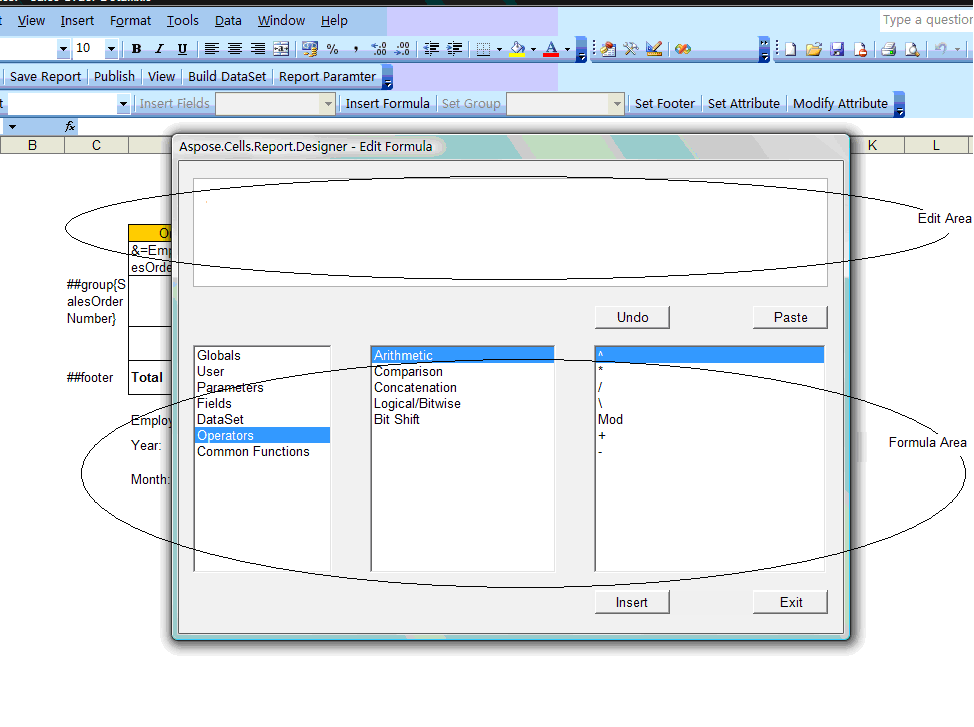# Formula Editor Window

Contents
[ ]

To edit a formula in a Microsoft Excel cell:

1. In Microsoft Excel, select a cell.
2. Open the Edit Formula dialog by clicking Edit Formula on the tool bar. (Adding Reporting Services Formulas walks through an example that edits a formula.) The dialog is divided into section: the edit area at the top, and the formula area at the bottom. Use the formula area to populate the edit area.
3. Select a category (user, parameters, fields etc.) from the Report Fields list (the left-hand list).
4. Select the type from the Functions list (in the middle).
5. Select an option from the Operators list (the right-hand list).
6. Click Insert to add the expression to the Edit area.
7. Click Insert when the expression is complete. The formula is inserted into the cell.

The Edit Formula dialogThe Edit Formula dialog is divided into sections, described below.

#### Edit Area

This is the area where you create or edit a formula. Create a formula by double-clicking on any of the components listed in the Report Fields, Functions or Operators lists. When you choose a component, the required syntax is also inserted. You can also manually enter a formula.

#### Formula Area

The formula area contains three sections, each listing information used to build a formula.

• Report Fields - the left-hand list lists all the database fields accessible for the report. It also lists any formulas or groups already created.
• Functions - the middle list contains functions, pre-built procedures that return values. They perform calculations such as AVERAGE, SUM, COUNT, SIN, UPPERCASE and so on.
• Operators - the “action verbs” used in formulas. Operators describe an operation or an action to take place between two or more values. Examples of operators: add, subtract, less than and greater than etc.

#### Controls

The dialog has several controls:

Button Name Description
Undo Undoes an action.
Paste Pastes a character string made up of the components listed in the formula area into the edit area.
Insert Takes the value in the edit area and inserts it as a formula into a cell.
Exit Closes the Formula Editor.